# Cesàro summation methods

(diff) ← Older revision | Latest revision (diff) | Newer revision → (diff)

A collection of methods for the summation of series of numbers and functions. Introduced by E. Cesàro  and denoted by the symbol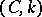.

A series(*)

with partial sumsis summable by the Cesàro method of order, or-summable, with sumif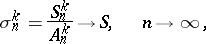where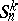and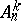are defined as the coefficients of the expansions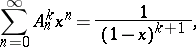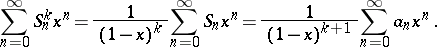Expressions forandcan be given in the formThe methodis a matrix summation method with matrix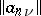,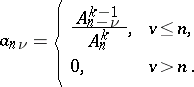Forthe method coincides with ordinary convergence, for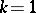it is the method of arithmetic averages. The methodsare totally regular for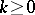and are not regular for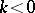. The power of the method increases asincreases: If a series is summable by the method, then it is summable with the same sum by the method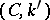for. This property does not hold for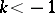. It follows from the summability of the series (*) by the methodthat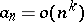. The methodis equivalent to and compatible with the summation methods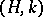of Hölder and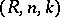of Riesz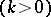(cf. Hölder summation methods; Riesz summation method). For anythe methodis weaker than Abel's method (cf. Abel summation method).

Originally, the methodswere defined by Cesàro for positive integer values of the parameter, and applied to the multiplication of series. They were later extended to arbitrary values of, including complex values. The methodshave numerous applications, e.g. to the multiplication of series, in the theory of Fourier series, and to other questions.

How to Cite This Entry:
Cesàro summation methods. Encyclopedia of Mathematics. URL: http://encyclopediaofmath.org/index.php?title=Ces%C3%A0ro_summation_methods&oldid=19282
This article was adapted from an original article by I.I. Volkov (originator), which appeared in Encyclopedia of Mathematics - ISBN 1402006098. See original article